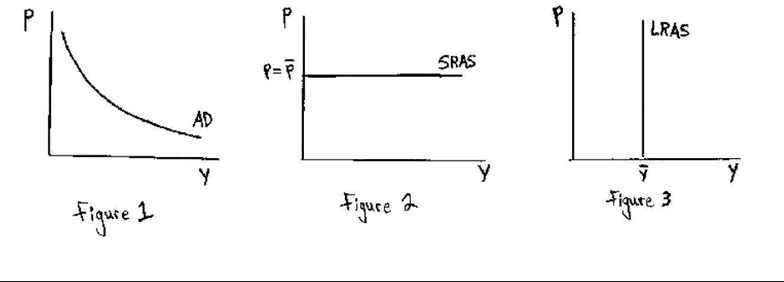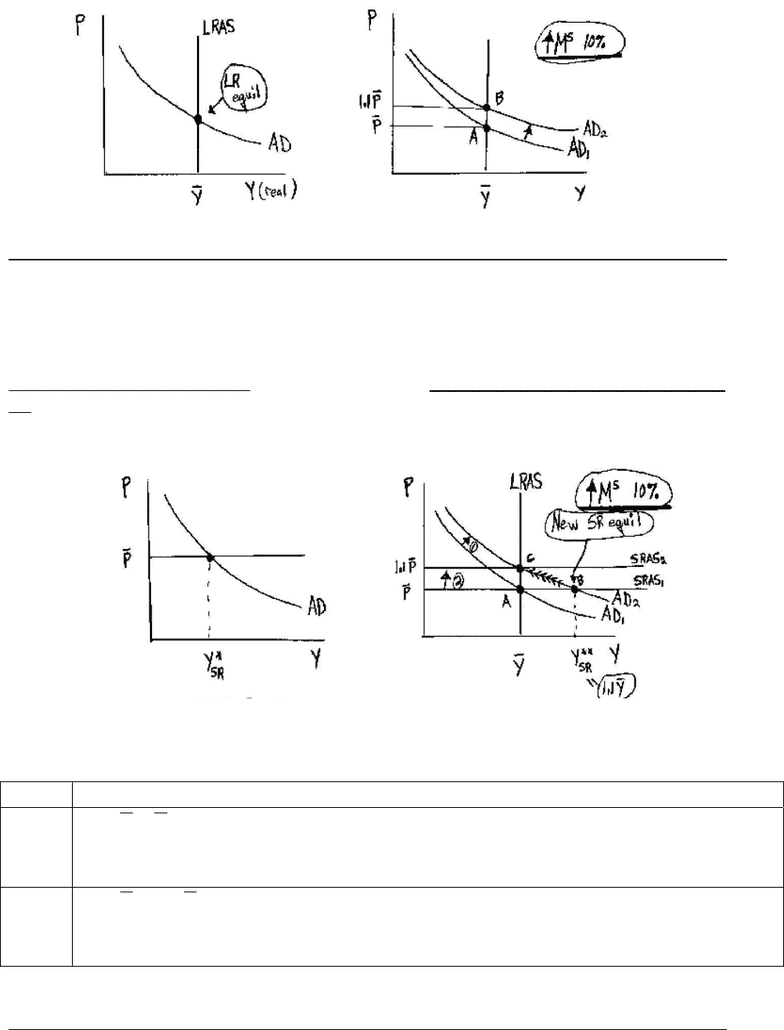# MGEB06H3 Lecture Notes - Lecture 9: Business Cycle, Stagflation, Nominal Rigidity

108 views7 pages
School
Course
ProfessorReview of AD/AS Model (Chapter 9)
Aggregate Demand Curve (AD Curve) - Shows the relationship between the aggregate
(nominal) price level and real aggregate output (GDP) demanded. The quantity equation, money
market equilibrium, can be employed to show the AD curve is downward sloping (see figure 1).
Short-Run Aggregate Supply Curve (SRAS Curve) - Shows the pairs of real GDP and the
(nominal) price level consistent with aggregate supply in the short-run (SR). Price is assumed to
be fixed in the short-run (i.e. the short-run is such a short period of time that price can not vary)
which implies that the SRAS Curve is horizontal at the fixed price level (see figure 2 below).
Note: This implies that in the SR firms and workers are willing to do things that are not optimal
in the long-run (such as hire in any amount of labour to satisfy demand in the SR).
Long-Run Aggregate Supply Curve (LRAS Curve) - Shows the pairs of real GDP and the
(nominal) price level consistent with aggregate supply in the long-run (LR). Since output is
determined solely by real factors (inputs and technology) in the long-run (recall chapter 3) real
GDP in the long-run is independent of the aggregate price level. Therefore, the LRAS Curve is
vertical at the amount of real GDP as determined by current inputs and technology (Ybar) as
represented below in figure 3.
Long-Run General Equilibrium (in the AD/AS Model) – The LR equilibrium is located
where the current AD Curve intersects the current LRAS Curve. In the LR output is supply
determined (i.e. in the LR prices vary to ensure Y = Ybar, so the LR P level is at the point where
the current AD curve intersects the current LRAS). Note: In the LR the level of output is
determined by the location of the LRAS curve (this location is fixed since inputs & technology
are fixed).
For example: Suppose that the (nominal) money supply (MS) is increased by 10%.
The Quantity Theory of Money (QTM) implies that the so called “quantity equation” must hold
(that is MV = PY) if both velocity (V) and the (nominal) price level (P) are held constant (in
the SR) then this implies that if M increases by 10% then Y must also increase (also by 10%).
This implies that the AD Curve shifts up towards the right (as seen below).
In the LR price is assumed to be perfectly variable and changes until AD is set equal to LRAS
(i.e. to restore LR equilibrium). The result is that, in the LR, there is no change in Y and all other
real variables (LR Neutrality of Money - note here none of Y, T, G, nor r (Fisher Effect) did not
change so nothing real changed in the LR) and we only get a LR proportionate increase in the
price level (i.e. the price level rises by 10% which is the same result we saw in Chapter 4).
Unlock document

This preview shows pages 1-2 of the document.
Unlock all 7 pages and 3 million more documents.

Already have an account? Log in2
Short-Run Equilibrium (in the AD/AS Model) – The SR equilibrium is located where the
current AD Curve intersects the current SRAS Curve. Now output is said to be demand
determined as the location of the AD curve is crucial. The location of the SRAS Curve may be
stuck in the SR, i.e. Sticky Prices.
If the prices are sticky in the SR then a 10% increase in the money supply is not neutral in the
SR. That is, the resulting new SR equilibrium is characterised by changes in one or more real
variables (for example, here real output Y, and all variables directly linked to it, change - see
point B).
In the long-run, price adjusts (rises here) and we return to the long-run equilibrium described
above (i.e. point C).
Effect of a 10% Increase in the MS (& the Neutrality of Money)
SR YPVM , since P & V are constant (in the SR) then if MS rises by
10% then so does real output (Y)
(i.e. money is NOT neutral in the SR)
LR YPVM , since Y & V are constant (in the LR) then if MS rises by
10% then so does the nominal price level (P)
(i.e. money is neutral in the LR – it only impacts nom vars)
Transition From SR Equilibrium to LR General Equilibrium - The economy moves
from point B to point C on its own over time (i.e. the economy is self-correcting). This occurs
because SR equilibrium output (recall that Y*SR = Yd, i.e. in the SR output is demand
determined) above (below) the full employment level of output (Ybar = natural level of output =
Unlock document

This preview shows pages 1-2 of the document.
Unlock all 7 pages and 3 million more documents.

Already have an account? Log in

# Get OneClass Notes+

Unlimited access to class notes and textbook notes.

75% OFF
\$8 USD/m
###### Monthly
\$30 USD/m
You will be charged \$96 USD upfront and auto renewed at the end of each cycle. You may cancel anytime under Payment Settings. For more information, see our Terms and Privacy.
Payments are encrypted using 256-bit SSL. Powered by Stripe.